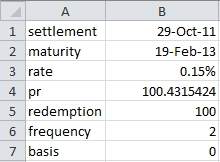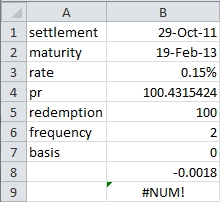## 11 financial calculations that you can’t do in EXCEL

Oct 18

Written by: Charles Flock
10/18/2011 8:16 PMHere is a list of 11 very simple financial calculations that you can’t do in EXCEL, either because the EXCEL design of a function doesn’t support the input data, or because EXCEL produces a result that is wrong  or unreliable. Of course, we used EXCEL 2010 and, of course, you can do them in SQL Server using XLeratorDB.
Part 1 of 11 - Enter a negative yield to calculate the price of a bond
This list is based, for the most part, on inquiries sent to us by XLeratorDB users, all of which asked one of two questions. The first is, “Why is your answer different than EXCEL?” The answer is, because the EXCEL answer is wrong. The second is, “How can I do this [what your function does] in EXCEL?” You can’t, unless you are willing to do a bunch of research, write a chunk of VBA code, test it, and then hope that you got everything right.
In this article, we are focused exclusively on EXCEL’s financial functions. We are not doing anything exotic or esoteric; we just try to calculate things like the price of a bond, interest amounts, internal rates of return, and periodic payments. What we found was the functions:
1.       are inconsistent with one another,
2.       do not capture the requisite date to calculate a correct result, and
3.       do not return the correct result
We are focused here on basic number-crunching. We are not interested in graphs, or in PowerPivot, anything other than looking at our ability to calculate values in EXCEL. The following list is in no particular order.
1. Enter a negative yield to calculate the price of a bond
Set up a worksheet with the following data.Enter this formula in B8: =YIELD(B1,B2,B3,B4,B5,B6,B7). Enter this formula in B9: =PRICE(B1,B2,B3,B8,B5,B6,B7). You should get the following result.The #NUM! error value in B9 is returned because the PRICE function will not accept a negative value for yield, even though the YIELD function calculated it. Normally, the value calculated by the PRICE function will equal the value for price supplied to the YIELD function. So, why does EXCEL have a rule that the yield cannot be negative?
Here’s the PRICE function in SQL Server, with the negative yield as input.
SELECT wct.PRICE(
'2011-10-29'     --settlement
,'2013-02-19'     --maturity
,0.0015           --rate
,-0.0018          --yld
,100              --redemption
,2                --frequency
,0                --basis
) as PRICE
This produces the following result.
PRICE
----------------------
100.431542431914
Here’s a SQL Server Example using the YIELD function as INPUT into the PRICE function, replicating what we should have been able to do in EXCEL.
SELECT wct.PRICE(
n.settlement
,n.maturity
,n.rate
,wct.YIELD(
n.settlement
,n.maturity
,n.rate
,100.431542431914
,n.redemption
,n.frequency
,n.basis)
,n.redemption
,n.frequency
,n.basis)
FROM (VALUES
('2011-10-29'
,'2013-02-19'
,0.0015
,100
,2
,0)
) n(settlement, maturity, rate, redemption,frequency, basis)
This produces the following result.
----------------------
100.431542431916
This limitation is also imposed by EXCEL in the ODDFYIELD and ODDLYIELD functions, but we will only count this as one item toward the total of 11, as we have other issues with those functions.

Tags:
Categories:
Location: BlogsThe WestClinTech Blog

## Search Blogs

 KeywordsPhrase

## Blog Archives

Archive
 < March 2023 >
SunMonTueWedThuFriSat
2627281234
567891011
12131415161718
19202122232425
2627282930311
2345678
Monthly
Go

### SupportCopyright 2008-2023 Westclintech LLC         Privacy Policy        Terms of Service